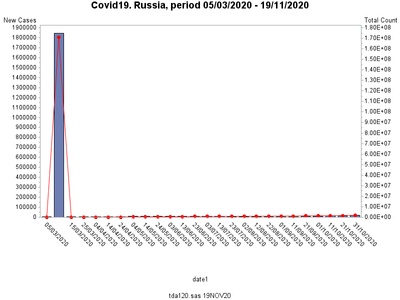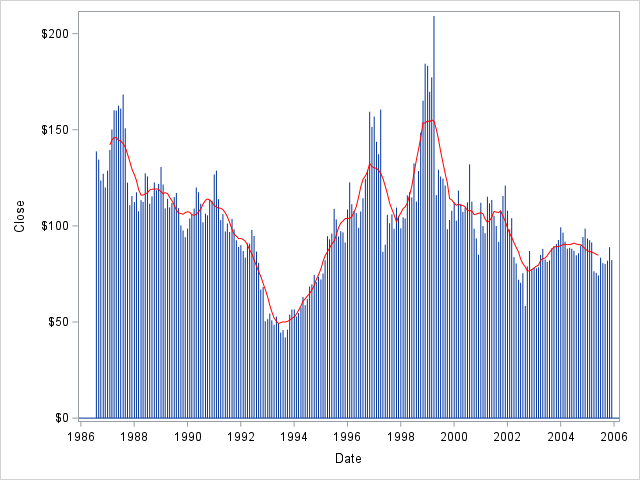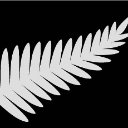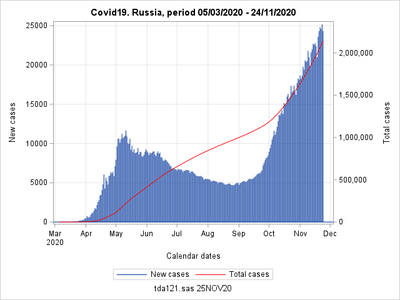## Gbarline procedure: how to make use of formatted values for tickmark labels

I am using proc gbarline  within IML. the following example code  produces the graph

proc gbarline data=dsin;
format date1;

symbol1 c=red value=dot;
axis1 label=("New Cases");
axis2 label=("Total Count");
axis3 value=(a=-45);
bar date1 / discrete sumvar=newca raxis=axis1 maxis=axis3 levels=all;
plot / sumvar=totca axis=axis2;
run;

The graph looks likeThe horizontal axe labels are too overlapped.

My idea is to label only some tickmarks, one way is to use SAS generated format, ususally I was using this approach in SAS graph procedures.. However in this case when I use the code

proc gbarline data=dsin;
format date1 date_fmt.;

symbol1 c=red value=dot;
axis1 label=("New Cases");
axis2 label=("Total Count");
axis3 value=(a=-45);
bar date1 / discrete sumvar=newca raxis=axis1 maxis=axis3 levels=all;
plot / sumvar=totca axis=axis2;
run;
quit;

The result is not what I would expect:I would appreciate any hints or statement, that this is not possible.

1 ACCEPTED SOLUTION

Accepted Solutions

## Re: Gbarline procedure: how to make use of formatted values for tickmark labels

The basic/fundamental problem is that bar charts generally treat each bar as a discrete evenly-spaced thing, and therefore want to label each bar. You're wanting the bar axis to be continuous, so that you can only label certain points along the axis.

In order to do that, I suggest using a 'needle' plot (rather than a bar chart), and then overlay the average line. You can do that with either SAS/Graph gplot, or with ODS Graphics sgplot. I will demonstrate below one way to do it with sgplot:

data my_data; set sashelp.stocks (where=(stock='IBM'));
run;
proc expand data=my_data out=my_data;
convert close=close12 / method=none transformout=(cmovave 12 trim 6);
run;
proc sgplot data=my_data noautolegend;
needle y=close x=date;
series y=close12 x=date / lineattrs=(color=red);
run;7 REPLIES 7ChrisNZ
Tourmaline | Level 20

## Re: IML Gbarline procedure: how to make use of formatted values for tickmark labels

Moved to Graphics community.

@Rick_SAS Any idea?

Here is how to replicate the behaviour:

``````data _F;
retain FMTTYPE 'N' FMTNAME 'mthstart' START '01jan1900'd ;
do until( START='01oct2100'd );
START=intnx('month',START,1);
END  =START;
LABEL=put(START,monyy7.);
output;
end;
HLO='O'; LABEL=' '; output;
run;
proc format cntlin=_F; run;

proc gbarline data=SASHELP.CITIDAY;
format DATE mthstart.;
vbar DATE / sumvar=SNYDJCM discrete levels=all;
plot      / sumvar=SNYSECM ;
run;``````

Also, how to remove WARNING: The intervals on the axis ... are not evenly spaced.  ?

## Re: IML Gbarline procedure: how to make use of formatted values for tickmark labels

It seems, i have been using the same structure, I have prepared format like
********************************start code:;
proc summary nway noprint data=dsin;
var date1;
output out=sum1 min=a_min max=a_max;
run;

data _null_; set _last_; length sa \$ 80;
file '_date_fmt.txt' lrecl=80;
put 'proc format; value date_fmt';
do a=a_min to a_max by 10;
if ((a_max-a)>=10) then do;
sa=put( a, 6.)!! '="'!! compress(put(a,ddmmyy10.))!!'"';
put sa \$char80.;
end;
end;
put 'other=" ";';
run;
%inc "_date_fmt.txt";run;
********************************end code:;

However this was not working properly. Still, I will check if your code is
working

## Re: IML Gbarline procedure: how to make use of formatted values for tickmark labels

SASHELP.CITIDAY

in my version of SAS9.4

However, I made some checking and have realized, that this is not working in my case.

The  specificity of the data set used in my case lies in the fact, that values are available for a continuous set of dates, while  only some of them should have tickmarks. Specially generated format provides empty (blank) labels for most of the dates, however when applied, such a format doesn't work in  proc gbarline. While working in SAS graph procedures. Later I will submit some example.ChrisNZ
Tourmaline | Level 20

## Re: IML Gbarline procedure: how to make use of formatted values for tickmark labels

Your question is clear and the behaviour has been reproduced.

I understand why this happens but cant think of a way around it, except using SAS/Graph. Maybe @DonH_sas would have an idea?

## Re: Gbarline procedure: how to make use of formatted values for tickmark labels

The basic/fundamental problem is that bar charts generally treat each bar as a discrete evenly-spaced thing, and therefore want to label each bar. You're wanting the bar axis to be continuous, so that you can only label certain points along the axis.

In order to do that, I suggest using a 'needle' plot (rather than a bar chart), and then overlay the average line. You can do that with either SAS/Graph gplot, or with ODS Graphics sgplot. I will demonstrate below one way to do it with sgplot:

data my_data; set sashelp.stocks (where=(stock='IBM'));
run;
proc expand data=my_data out=my_data;
convert close=close12 / method=none transformout=(cmovave 12 trim 6);
run;
proc sgplot data=my_data noautolegend;
needle y=close x=date;
series y=close12 x=date / lineattrs=(color=red);
run;## Re: Gbarline procedure: how to make use of formatted values for tickmark labels

Thanks,

yes, I have arrived to these options too. As well as I have found one my graph with bars produced using annotate. This provides more control, though requires some more complicated  code.

## Re: Gbarline procedure: how to make use of formatted values for tickmark labels

After all, I have arrived to acceptable  solution:

data dsin; set &covidin Nobs=nobs;
run;

proc summary nway noprint data=dsin;
var date1;
output out=sum1 min=a_min max=a_max;
run;

data _null_; set _last_;
call symput('period', put(a_min,ddmmyy10.)!!' - '!!put(a_max,ddmmyy10.));
run;
title "Covid19. Russia, period &period.";
proc sgplot data=dsin;
format totca comma.;
needle y=newca x=date1;
series y=totca x=date1 /y2axis lineattrs=(color=red);
xaxis grid;
Yaxis grid;
Y2axis offsetmax=0.1;
Label newca="New cases"
date1="Calendar dates"
totca="Total cases";
run;Discussion stats
• 7 replies
• 718 views
• 3 likes
• 3 in conversation